Do you like the article?
Share it with others -

Use new possibilities of MetaTrader 5

# MetaTrader 5 and MATLAB Interaction

5 August 2010, 10:22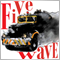5
27 639

### Introduction

My first article Interaction between MetaTrader 4 and MATLAB Engine (Virtual MATLAB Machine) was noticed by MQL-community. Some readers (1Q2W3E4R5T) were even able to move this project from Borland to VS2008. But time runs forward relentlessly and (sad but true) MetaTrader 4 is disappearing, giving way to its successor MetaTrader 5 with MQL5, that introduced pointers and dynamic memory.

Thanks to these innovations, we have the opportunity to write a universal library of interaction with MATLAB Engine virtual machine, and to directly link libraries, generated by MATLAB, with MetaTrader 5. This article covers such a functionality. This article logically continues the previous and more thoroughly covers the problem of interaction between MetaTrader 5 and MATLAB.

To make the scope of this article more understandable to unprepared readers, we will divide it into three parts: theory, reference and practice. Theory will cover the types of data used in MQL5 and MATLAB, as well as their mutual conversion. In Reference you will learn the linguistic structures and syntax of functions, needed to create a DLL. And in Practice we will analyze "pitfalls" of this interaction.

Experienced readers can skip Theory and Reference, and start with Practice. Others are urged to read Theory and Reference, and only then proceed to Practice. Also it's worth to read books mentioned in the "Literature" section.

### 1. Theory

1.1 Data Types in MATLAB and MQL5

1.1.1 Simple Data Types

Let's proceed.

First of all, we need to get acquainted with the inner worlds of MQL5 and MATLAB. After perfunctory inspection of variable types, we conclude that they are almost identical:

MQL5
Size in bytes
Minimal value
Maximal value
MATLAB
char
1
-128
127
Array int8/char
uchar
1
0
255
Array int8/char
bool
1
0(false)
1(true)
Array logical
short
2
-32768
32767
Array int16
ushort
2
0
65535
Array int16
int
4
-2147483648
2147483647
Array int32
uint
4
0
4294967295
Array int32
long 8
-9223372036854775808
9223372036854775807 Array int64
ulong 8
0
18446744073709551615
Array int64
float 4
1.175494351e-38
3.402823466e+38
Array single
double
8
2.225073858507201e-308
1.7976931348623158e+308
Array double

Table 1. Data Types in MATLAB and MQL5

There is one major difference: the variables in MQL5 can be simple or composite (complex), and in MATLAB all variables are multidimensional (complex) - i.e. matrix. You must always remember about this difference!

### 1.1.2 Complex Data Types

In MQL5 there are 4 complex types of data: arrays, strings, structures and classes. Complex data type is set of several simple data types, combined into memory block of certain length. When dealing with such data you always need to know either memory block size in bytes, or number of elements (except classes). We are interested only in arrays and strings, because submitting classes and MQL5 structures to MATLAB makes no sense.

When passing arrays of any type you need to know: type (dimension) and number of elements using the ArraySize() function. Particular attention should be given to indexing in MetaTrader 5 - usually it is backwards (i.e. the first element contains more recent data than the next). Check this using the ArrayIsSeries() function. And MATLAB has the following indexing: the first element contains the older data than the next - so you must "reverse" your arrays before sending them to MATLAB, if flag AS_SERIES = TRUE. Based on the above, let's agree with the following:

• "Reverse" arrays "invisibly" to MQL5-programs, except for arrays of the char type and 2-dimensional arrays - leave them unchanged.
• Reverse "invisibly" all arrays from MATLAB, and assign the AS_SERIES flag with TRUE, except for arrays of the char type and 2-dimensional arrays - leave them unchanged.
• In every array in MQL5-program, created according to "backwards" indexation, the AS_SERIES flag must be TRUE, except for arrays of the char type and 2-dimensional arrays - leave them unchanged.

But this is not the only limitation when working with arrays. When you work with multidimensional arrays, or matrices to be more correct, especially from MATLAB, we introduce the restriction for no more than 2 dimensional arrays. Here the AS_SERIES flag can not be TRUE, and therefore such arrays are not "reversed".

Don't forget that strings in MQL5 are not arrays of the char type elements. So when passing strings comes a slight problem: in MQL5 strings encoded using Unicode, and MATLAB uses ANSI encoding. So before you pass a string, it should be converted into array of ANSI characters using the StringToCharArray() function. And vice versa, when you get a characters array from MATLAB, convert it using the CharArrayToString() function (see Table 2). To avoid confusion, agree: store all strings in MQL5-programs using Unicode, no arrays of the char type.

1.2 Comparison of MQL5 and MATLAB Data Types

In order to reduce quantity of functions and to simplify library algorithm, we will reduce quantity of types by means of automatic conversion, that should not affect the integrity of data. The following table illustrates the rule of data type conversion from MQL5 into MATLAB:

MQL5
MatLab equivalent
char
uchar
Array char
bool
Array logical
short
ushort
int
uint
Array int32
long
ulong
Array int64*
float
double
Array double
string
Array char, using the StringToCharArray() <=> CharArrayToString() functions

* With this type of conversion there is a loss of accuracy. We will not use it, but you can use such conversion in your programs.

Table 2. Comparison of MQL5 and MATLAB Data Types

Now you are familiar with data types used in MQL5 and MATLAB. You know what "pitfalls" await in data passing and how to bypass them competently. You still have to know MATLAB Engine API and become familiar with MATLAB Compiler 4.

### 2. MATLAB Engine API Reference, MATLAB Compiler 4 Reference and C++ Input/Output Library Reference

This section introduces you to the most important functions of MATLAB Engine API, features of MATLAB Compiler 4 and number of useful functions of C++ standard input/output library. So, let's begin.

2.1 MATLAB Engine API and MCR Functions

MATLAB Engine - is external interface that enables other programs to use MATLAB desktop. It provides a fully functional work of all MATLAB packages without any restrictions.

Although it is not said in the documentation, but in terms of system programmer - it's just a virtual machine, like PHP, MySQL, etc. that supports a simple and relatively quick way to exchange data between MetaTrader 4/5 and MATLAB.

This method of connecting external programs with MATLAB package is recommended by developers. The interface consists of six functions:

Engine *pEng = engOpen(NULL) — this function calls MATLAB desktop, the parameter is always NULL, returns a pointer to the desktop descriptor.

int exitCode = engClose(Engine *pEng) — this function closes desktop, returns number of remaining users of MATLAB desktop, where:

• Engine *pEng — pointer to the desktop descriptor.

mxArray *mxVector = mxCreateDoubleMatrix(int m, int n, int ComplexFlag) — this function creates a variable (matrix) of MATLAB desktop, returns a pointer to variable (matrix), where:

• mxArray *mxVector — pointer to matrix variable.
• int m — number of rows.
• int n — number of columns.
• ComplexFlag — type of complex number, for MetaTrader 4/5 mxREAL.
void = mxDestroyArray(mxArray *mxVector) — this function destroys MATLAB matrix, it is needed to clear memory, where:
• mxArray *mxVector — pointer to matrix variable.
int = engPutVariable(Engine *pEng, char *Name, mxArray *mxVector) — this function sends variable to desktop. You must not only create variables of the mxArray type, but also send them to MATLAB, where:
• Engine *pEng — pointer to the desktop descriptor.
• char *Name — variable name of the char type in MATLAB desktop.
• mxArray *mxVector — pointer to matrix variable.
mxArray *mxVector = engGetVariable(Engine *pEng, char *Name) — this function gets variable from desktop - the inverse of the previous function. Only variables of the mxArray type are accepted, where:
• mxArray *mxVector — pointer to matrix variable.
• Engine *pEng — pointer to the desktop descriptor.
• char *Name — variable name of the char type in MATLAB desktop.
double *p = mxGetPr(mxArray *mxVector) — this function gets pointer to array of values, it is used to copy data along with memcpy() (see 2.3 C++ Standard Input/Output Library), where:
• double *p — pointer to array of the double type.
• mxArray *mxVector — pointer to matrix variable.
int = engEvalString(Engine *pEng, char *Command) — this function sends commands to MATLAB desktop, where:
• Engine *pEng — pointer to the desktop descriptor.
• char *Command — command for MATLAB, string of the char type.

You probably noticed that the MATLAB Engine API allows you to create mxArray structure only for the double type. But this restriction does not affect your possibilities, but will affect the algorithm of your library.

MCR (MCR instance) — is the special library of MATLAB package, that enables to run standalone applications/public libraries, generated by MATLAB environment on any computer. Note, that even if you have a complete MATLAB package, you still need to install the MCR library by running MCRInstaller.exe file, located in the <MATLAB>\Toolbox\compiler\deploy\win32 folder. So, before calling any public library function, created by MATLAB environment, you need to call MCR initialization function:

bool = mclInitializeApplication(const char **option, int count) – returns TRUE if MCR start was successful, otherwise FALSE, where:

• const char **option — string of options, like in mcc - R; usually is NULL
• int count — size options string, typically 0.

On ending public library work you must call:
bool = mclTerminateApplication(void) — returns TRUE if MCR was successfully closed.

2.2 MATLAB Compiler 4

MATLAB Compiler lets you to create the following from M-functions:

• Standalone applications that run even if MATLAB is not installed.
• C/C++ share libraries, that can be used without MATLAB on end-user systems.

Compiler supports most of the commands and packages of MATLAB, but not all. Full list of restrictions can be found on MATLAB website. This method allows you to create "software-independent bundle" of MetaTrader 5 and MATLAB, but in contrast to the MATLAB Engine, requires a well trained programmer and deep knowledge of compilation.

MATLAB Compiler requires at least one of the following C/C++ compilers:

• Lcc C (usually comes with MATLAB). It's only C compiler.
• Borland C++ versions 5.3, 5.4, 5.5, 5.6.
• Microsoft Visual C/C++ versions 6.0, 7.0, 7.1.

MATLAB Compiler 4, in contrast to its predecessors, generates only the interface code (wrapper), i.e. does not translate m-functions into binary or C/C++ code, but it creates a special file based on Component Technology File (CTF) technology, that includes integrations of various packages, required to support m-functions. MATLAB Compiler also encrypts this file with unique (unrepeated) 1024-bit key.

Now let's consider the algorithm of MATLAB Compiler 4 work, since ignorance of this topic will lead to many stupid mistakes at compilation time:

1. Dependencies analysis — at this stage determine all functions, MEX-files and P-files, that compiled m-functions depend on.
2. Creating archive - CTF-file is created, it is encrypted and compressed.
3. Generating the object code of wrapper – at this stage all source codes are created, needed for component:
• C/C++ interface code for m-functions specified in the command line (NameFile_main.c).
• Component file (NameFile_component.dat), which contains all the information needed to execute m-code (including encryption keys and paths, stored in CTF-file).
4. C/C++ translation. At this stage C/C++ source code files are compiled into object files.
5. Linking. The final stage of project building.

Now, when you are familiar with MATLAB Compiler algorithm behavior, you have to learn more about keys in order to have a detailed plan of actions, when using compiler (mcc):

Key
Purpose
a filename
Add the <filename> file to archive, determine what files will be added to the CTF archive
l
Macro, that generates a library of functions
N
Clear all paths, except the minimal necessary set of directories
p <directory>
Add path of translation according to procedure. Requires the -N key.
R -nojvm
Cancel MCR option (MATLAB Component Runtime, see MATLAB Help)
W
Manage creation of function wrappers
lib
Create initialization and completion functions
main
Create POSIX shell of the main() function
T
Specify the output stage
codegen
Create wrapper code for standalone application
compile:exe
Same as codegen
compile:lib
Create wrapper code for public DLL

Table 3. Keys of Matlab mcc Compiler (version 4)

Table 3 contains basic keys that may be useful in solving typical problems. For more help, use MATLAB commands help mcc or doc mcc.

We have to get acquainted with MATLAB linker, below are the main keys (mbuild):

Key
Purpose
-setup
In interactive mode, definition of compiler options file to use by default in future calls of mbuild
-g
Create program with debugging information. Append DEBUGFLAGS to the end of file.
-O
Optimization of object code

Table 4. Matlab mbuild Linker (version 4) keys

Table 4 lists the main keys. For more information use the help mbuild or doc mbuild commands.

2.3 C++ Standard Input/Output Library

Using the Standard Input/Output Library provides the correct data copying. Its use will save you from "stupid" errors that arise during the program design phase (for example: many novice programmers copy only pointer to memory block instead of copying the entire memory block). From entire Input/Output Library we are interested only in one function:

void *pIn = memcpy(void *pIn, void *pOut, int nSizeByte) – this function copies (clones) variable/array from pOut to pIn with size of nSizeByte bytes, where:

• void *pIn — pointer to array, where to copy.
• void *pOut — pointer to array, from which copying is made.
• int nSizeByte — the size of copied data, should not exceed the size of the pIn array, otherwise memory access error will occur.

### 3. Practice

Now we are done with theory and we can proceed with realization of MetaTrader 5 & MATLAB interaction.

As you probably guessed, this will be done in two ways: using MATLAB Engine virtual machine and using libraries generated by MATLAB Compiler. First, consider a simple, fast and versatile way of interaction — via MATLAB Engine.

This part of article must be read from beginning to end, as, despite the seeming difference between methods of interaction, they have one philosophy and familiar syntax of language constructs, and to learn something new is easier with simple examples.

3.1 Developing Universal Library of MetaTrader 5 & MATLAB Engine Interaction

This method of interaction can't be called elegant and fast, but it is the most reliable and covers the entire MATLAB package. Of course, we should mention the speed of final model development. The essence of development is to write a universal library-wrapper for MetaTrader 4/5 & MATLAB Engine interaction. After this MetaTrader 4/5 script/indicator/expert can manage MATLAB virtual desktop. And the entire mathematical algorithm may be stored in MQL-program as strings, so you can use it to protect your intellectual property (for more details see the "Protect Yourselves, Developers!" article). It also may be stored in m-functions or P-functions separate files in the <MetaTrader 5>\MQL5\Libraries folder.

Possible areas of application of such interaction:

• To test or demonstrate "mathematical models/ideas" without having to write complex programs (protection of intellectual property can be arranged as in MQL-program and by means of MATLAB package - using P-functions).
• To write complex mathematical models using all features of MATLAB.
• To all those who are not going to distribute their scripts/indicators/experts.

Let's proceed. I hope that you have read the 1.1 Data types in MATLAB and MQL5, 1.2 Comparison of MQL5 and MATLAB Data Types, 2.1 MATLAB Engine API and MCR Functions and 2.3 C++ Standard Input/Output Library sections, as we will not pause and analyze them anymore. Carefully read the following block-scheme, that illustrates the algorithm of future library: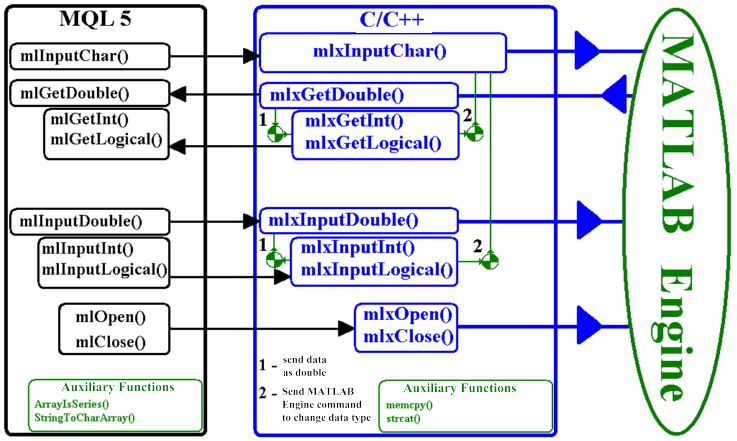Figure 1. Block-Scheme of Library Algorithm

As seen on Figure 1, the library consists of three main blocks. Consider their purposes:

• MQL5 block, preliminary preparation of sent/received data:
• Reverse arrays.
• Types conversion.
• Strings encodings conversion.
• C/C++ block:
• Converts array into the mxArray structure.
• Passes MATLAB Engine commands.
• MATLAB Engine block — calculations system.

Now, let's deal with algorithms. We'll begin with MQL5 block. Attentive reader has already noticed that it will focus on the implementation of what was written in the Data Types in MATLAB and MQL5 section. If you've missed it, you'll hardly understand why all this is necessary.

Algorithm of the mlInput <variable_type> functions is almost identical. Let's discuss its work using the mlInputDouble() function that provides input of variables of the double type to MATLAB virtual machine.

Here is the prototype:

bool mlInputDouble(double &array[],int sizeArray, string NameArray), where:

• array — reference to variable or array of the double type.
• sizeArray — array size (number of elements, not bytes!).
• NameArray — string, containing unique variable name for MATLAB virtual machine (name must correspond to MATLAB requirements).

Algorithm:

1. Convert NameArray string to char array using the StringToCharArray() function.
2. Check the type of indexing using the ArrayIsSeries() function. If the type of indexing is normal — pass value to the mlxInputDouble() function.
ELSE indexing of timeseries array:
"reverse" array and pass value to the mlxInputDouble() function.
3. End function, pass the returned value to the mlxInputDouble() function.

Algorithm of the mlGet <variable_type> functions is also almost identical. Let's discuss its work using the mlGetDouble() function, that returns variable of the double type from MATLAB virtual machine.

The prototype:

int mlGetDouble(double &array[],int sizeArray, string NameArray), where:

• array — reference to variable or array of the double type.
• sizeArray — array size (number of elements, not bytes!).
• NameArray — string, containing unique variable name for MATLAB virtual machine.

Algorithm:

1. Convert NameArray string to char array using the StringToCharArray() function.
2. Find the size of array using the mlxGetSizeOfName() function.
• IF size is MORE THAN ZERO, allocate the recipient array of needed size using the ArrayResize() function, get data of mlxGetDouble(), return array size.
• IF size is ZERO, return error, i.e. null value.

That's it! The mlGetInt() and mlGetLogical() functions produce "shadow" conversion of types double ->; int/bool. For this purpose these functions create a temporary memory buffer in their bodies. This is a forced measure, because unfortunately MATLAB API does not allow to create mxArray structures for data types other than double. However, this does not mean that MATLAB operates exclusively the double types.

C/C++ block is far easier - it should provide data translation from the double type into the mxArray structure. It is done using the mxCreateDoubleMatrix(), mxGetPr() and memcpy() functions. Then, using the engPutVariable() function it passes data to MATLAB virtual machine, and to extract data it uses the engGetVariable() function. Again, pay attention to functions with prefixes Int and Logical — as seen in the block-scheme, they don't directly interact with MATLAB, but use the mlxInputDouble/mlxGetDouble and mlxInputChar() functions. Algorithm of their behavior is simple: call of the mlxInputDouble/mlxGetDouble function — input/output values as double(!) and send the "shadow" MATLAB command to convert data type via the mlxInputChar() function.

MATLAB Engine block is even easier. It provides only mathematical functions. Its behavior depends on your commands and your m/p-functions.

Now, when all the "details" of the project are clear, it's time to deal with project building.

Any such build begins with the creation of main library — in our case it is C/C++ block. For this purpose, in any ANSI-text editor (Notepad, Bred, etc.) create a file with the DEF extension. It is desirable that the name of this file consist of Latin characters with no spaces and punctuation, otherwise you will "hear" many flattering "words" from your compiler... This file provides the permanence of your functions. If this file is absent, C/C++ compiler will invent his own "exotic names" to export functions.

This file contains: LIBRARY — control word, LibMlEngine — name of the library, and EXPORTS — second control word, then come the names of functions. As you probably knew, the names of export functions can't have spaces and punctuation. Here is the text of the DllUnit.def file from MATLABEngine.zip archive:

LIBRARY LibMlEngine
EXPORTS
mlxClose
mlxInputChar
mlxInputDouble
mlxInputInt
mlxInputLogical
mlxGetDouble
mlxGetInt
mlxGetLogical
mlxGetSizeOfName
mlxOpen

So, we have the first file of project. Now open Windows Explorer and go to the '<MATLAB>\Extern\include' folder. Copy the engine.h file (header file of MATLAB virtual machine) to folder, where you project is built (if you won't do this, you will have to manually specify the path to file at the stage of compilation).

Now it's time to create C/C++ block. We will not include the entire source code of program in the article, because this file can be found in MATLABEngine.zip as DllUnit.cpp and it is well commented. Note that it's better to create functions using __stdcall convention — i.e. parameters are passed through the stack, and function cleans the stack. This standard is "native" for Win32/64 API.

Consider how to declare a function:

extern "C" __declspec(dllexport) <variable_type> __stdcall Function(<type> <name>)

1. extern "C" __declspec(dllexport) — tells C++ compiler that function is external.
2. <variable_type> — type of returned variable, may be: void, bool, int, double, composite types (known not only to Dll, but also to calling program) and pointers.
3.  __stdcall — declaration about passing parameters to function and back, it's a standard for Win32/64 API.
4. Funcion — your function name.
5. <type> <name> — type and name of input variable, maximal number of variables is 64.

This topic is covered in details in the How to Exchange Data: A DLL for MQL5 in 10 Minutes article.

C/C++ block building: for this you need to include standard input/output library and add to project the following files (in your compiler: Project->Add Project):

1. DllUnit.def
2. In <MATLAB>\Extern\lib\<win32/64>\<compiler>\ folder, where:
<MATLAB> — MATLAB main folder.
<win32/64> — either win32 folder for 32-bit OS, or win64 for 64-bit OS.
<compiler> — the "borland" folder for Borland C/C++ ver. 5-6, the "microsoft" folder for Microsoft Visual C++:
• libeng.lib
• libmx.lib

A common question like this may arise: "I have different version of compiler or no such a compiler in the list! (Very rarely there are no such files)". Let's see how to manually create a public library. We will consider how it's done in Visual C++ and in Borland C++:

1. In FAR open <MATLAB>\Bin\<win32/64> folder, where:
<MATLAB> — MATLAB main folder.
<win32/64> — either win32 folder for 32-bit OS, or win64 for 64-bit OS.
2. For Borland C++ enter: implib libeng.lib libeng.dll. The same for libmx.dll.
3. For Visual C++ enter: lib libeng.dll. The same for libmx.dll.
4. If other compiler: any compiler of any programming language must have this utility - Library Manager, usually this is a console program <compiler _folder>\bin\*lib*.exe.

By the way, I forgot to warn you - don't try to make 64-bit LIB for 32-bit compiler. First, find out if there is 64-bit addressing support in compiler help. If not, either looking for 32-bit MATLAB DLL, or choose another C/C++ compiler. Getting down to compilation, after which we get a library, that should be placed in the terminal_folder\MQL5\Libraries folder.

Now let's begin with MQL block. Run MetaEditor, click "New" and do as on following figures: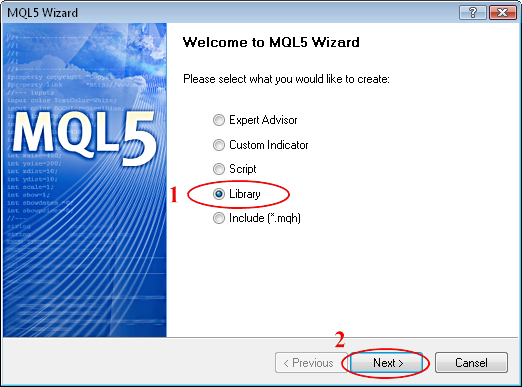Figure 2. MQL5 Wizard: Create Library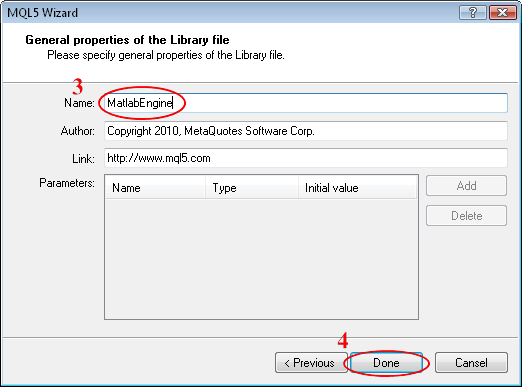Figure 3. MQL5 Wizard: General Properties of Library

Now, when Wizard MQL5 has created a template, proceed to its editing:

1. Describe functions import:

```//+------------------------------------------------------------------+
//| DECLARATION OF IMPORTED FUNCTIONS                                 |
//+------------------------------------------------------------------+
#import "LibMlEngine.dll"
void   mlxClose(void);                        //void – means: don't pass any parameters!
bool   mlxOpen(void);                         //void – means: don't pass and don't receive any parameters!
bool   mlxInputChar(char &CharArray[]);       //char& CharArray[] – means: pass a reference!
bool   mlxInputDouble(double &dArray[],
int sizeArray,
char &CharNameArray[]);
bool   mlxInputInt(double &dArray[],
int sizeArray,
char &CharNameArray[]);
bool   mlxInputLogical(double &dArray[],
int sizeArray,
char &CharNameArray[]);
int    mlxGetDouble(double &dArray[],
int sizeArray,
char &CharNameArray[]);
int    mlxGetInt(double &dArray[],
int sizeArray,
char &CharNameArray[]);
int    mlxGetLogical(double &dArray[],
int sizeArray,
char &CharNameArray[]);
int    mlxGetSizeOfName(char &CharNameArray[]);
#import
```

Note that MQL 5 you can pass "pointers" in two ways:

• void NameArray[] ;   // This method of passing from array allows only to read data. However, if you try to use this reference to "edit its contents", you'll get memory access error (in the best case for you, MetaTrader 5 will quietly handle the error in the SEH-frame, but we HAVEN'T WRITE a SEH-frame, so we can even miss the reason of error).
• void& NameArray[] ; // This method of passing allows you to read and edit array contents, but you must retain array size.

If function doesn't accept or doesn't pass parameters, always specify the void type.

2. We won't describe all functions of the MQL block, because you can find MatlabEngine.mq5 source code in MATLABEngine.zip.

Therefore, we'll consider the details of declaration and definition of external functions in MQL5:

```bool mlInputChar(string array)export
{
//... body of function
}
```

As seen in the example, the declaration and definition of function are combined. In this case, we declare a function named mlInputChar() as external (export), which returns value of the bool type and accepts the array string as parameter. Now compile ...

Now that we have completed the last block of the library and compiled it, it's time to test it in real conditions.

To do this, write a simple test script (or take it from MATLABEngine.zip, file: TestMLEngine.mq5).

Script code is simple and well commented:

```#property copyright "2010, MetaQuotes Software Corp."
#property version   "1.00"
#import "MatlabEngine.ex5"
bool mlOpen(void);
void mlClose(void);
bool mlInputChar(string array);
bool mlInputDouble(double &array[],
int sizeArray,
string NameArray);
bool mlInputInt(int &array[],
int sizeArray,
string NameArray);
int mlGetDouble(double &array[],
string NameArray);
int mlGetInt(int &array[],
string NameArray);
bool mlInputLogical(bool &array[],
int sizeArray,
string NameArray);
int mlGetLogical(bool &array[],
string NameArray);
int mlGetSizeOfName(string strName);
#import
void OnStart()
{
// Dynamic buffers for MATLAB output
double dTestOut[];
int    nTestOut[];
bool   bTestOut[];
// Variables for MATLAB input
double dTestIn[] = {   1,     2,    3,     4};
int    nTestIn[] = {   9,    10,   11,    12};
bool   bTestIn[] = {true, false, true, false};
int nSize=0;
// Variables names and command line
string strComm="clc; clear all;"; // command line - clear screen and variables
string strA     = "A";            // variable A
string strB     = "B";            // variable B
string strC     = "C";            // variable C
/*
** 1. RUNNING DLL
*/
if(mlOpen()==true)
{
}
else
{
mlClose();
return;
}
/*
** 2. PASSING THE COMMAND LINE
*/
if(mlInputChar(strComm)==true)
{
printf("Command line has been passed into MATLAB");
}
else printf("ERROR! Passing the command line error");
/*
** 3. PASSING VARIABLE OF THE DOUBLE TYPE
*/
if(mlInputDouble(dTestIn,ArraySize(dTestIn),strA)==true)
{
printf("Variable of the double type has been passed into MATLAB");
}
else printf("ERROR! When passing string of the double type");
/*
** 4. GETTING VARIABLE OF THE DOUBLE TYPE
*/
if((nSize=mlGetDouble(dTestOut,strA))>0)
{
int ind=0;
printf("Variable A of the double type has been got into MATLAB, with size = %i",nSize);
for(ind=0; ind<nSize; ind++)
{
printf("A = %g",dTestOut[ind]);
}
}
else printf("ERROR! Variable of the double type double hasn't ben got");
/*
** 5. PASSING VARIABLE OF THE INT TYPE
*/
if(mlInputInt(nTestIn,ArraySize(nTestIn),strB)==true)
{
printf("Variable of the int type has been passed into MATLAB");
}
else printf("ERROR! When passing string of the int type");
/*
** 6. GETTING VARIABLE OF THE INT TYPE
*/
if((nSize=mlGetInt(nTestOut,strB))>0)
{
int ind=0;
printf("Variable B of the int type has been got into MATLAB, with size = %i",nSize);
for(ind=0; ind<nSize; ind++)
{
printf("B = %i",nTestOut[ind]);
}
}
else printf("ERROR! Variable of the int type double hasn't ben got");
/*
** 7. PASSING VARIABLE OF THE BOOL TYPE
*/
if(mlInputLogical(bTestIn,ArraySize(bTestIn),strC)==true)
{
printf("Variable of the bool type has been passed into MATLAB");
}
else printf("ERROR! When passing string of the bool type");
/*
** 8. GETTING VARIABLE OF THE BOOL TYPE
*/
if((nSize=mlGetLogical(bTestOut,strC))>0)
{
int ind=0;
printf("Variable C of the bool type has been got into MATLAB, with size = %i",nSize);
for(ind=0; ind<nSize; ind++)
{
printf("C = %i",bTestOut[ind]);
}
}
else printf("ERROR! Variable of the bool type double hasn't ben got");
/*
** 9. ENDING WORK
*/
mlClose();
}
```

As seen from the script, we are entering values, and then get values. However, in contrast to MetaTrader 4, where we needed to know the size of buffer at design stage, in MetaTrader 5 it's not needed, as we use dynamic buffers.

Now that you've finally understood MATLAB virtual machine, you can begin using DLL built in MATLAB environment.

3.2 Technical guidelines of building/using DLL generated by MATLAB Compiler 4

In the previous section you've learned how to create a library for universal interaction with MATLAB package. However, this method has one drawback - it requires MATLAB package from end user. This restriction creates a number of difficulties in distribution of finished software product. That's why MATLAB mathematical package has a built-in compiler, that allows you to create "standalone applications" independent from MATLAB package. Let's take a look at it.

For example, consider a simple indicator - moving average (SMA). Slightly upgrade it by adding a Neural Network Filter (GRNN), that allows to smooth "white noise" (random bursts). Name the new indicator as NeoSMA, and filter as GRNNFilter.

Thus we have two m-functions, of which we want to create a DLL, that can be called from MetaTrader 5.

Now remember that the MetaTrader 5 searches fro DLLs in following folders:

• <terminal_dir>\MQL5\Libraries
• <terminal_dir>
• Current folder
• System folder <windows_dir>\SYSTEM32;
• <windows_dir>
• Directories listed in the system environment variable PATH.

Therefore, place into one of these directories two m-functions (NeoSMA.m and GRNNFilter.m), where we will build DLL. I draw your attention to this fact of placement, as this is done not by accident. Attentive reader already knows the MATLAB compiler feature - it preserves the paths when compiling (see "2.2 MATLAB Compiler 4").

Before you begin to compile project, you must configure compiler. To do this, follow these steps:

1. In MATLAB command line enter: mbuild -setup
2. Press 'y' to confirm find of C/C++ compatible compilers installed in your system.
3. Choose standard Lcc-win32 C compiler.
4. Press 'y' to confirm selected compiler.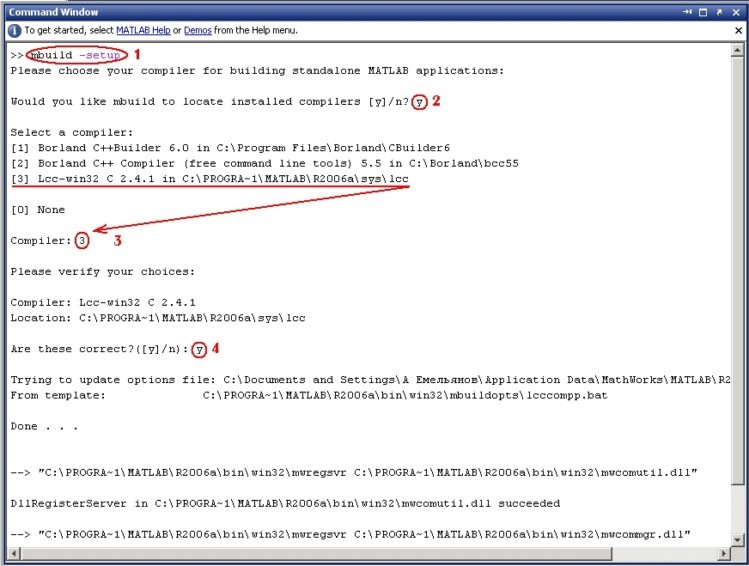Figure 4. Compiling the project

Now we are ready to move to the m-functions compilation process.

For this enter:

mcc -N -W lib:NeoSMA -T link:lib  NeoSMA.m GRNNFilter.m

Explain the keys:

-N                                     —  to skip all unnecessary paths
-W lib:NeoSMA                   —  tells compiler that NeoSMA is the name of library
NeoSMA.m and GRNNFilter.m  —  m-functions names

Now, let's see what compiler has created:

• mccExcludedFiles.log  —  log-file containing compilers actions
• NeoSMA.c  —  C version of library (contains С-code of wrapper)
• NeoSMA.ctf  —  CTF file (see 2.2 MATLAB Compiler 4) section
• NeoSMA.h  —  header file (contains declarations of libraries, functions, constants)
• NeoSMA.obj  —  object file (source file containing machine and pseudo code)
• NeoSMA.exports  —  exported functions names
• NeoSMA.dll  —  Dll for further linking
• NeoSMA.lib  —  Dll to use in C/C++ projects
• NeoSMA_mcc_component_data.c  —  C version on component (used for compliance with CTF-file, contains paths, etc.)
• NeoSMA_mcc_component_data.obj  —  object version of component (source file containing machine and pseudo code);

So let's handle with DLL, precisely with its internal structure. It consists of (basic functions only) from:

1. Main function of any DLL - BOOL WINAPI DllMain(), which (according to Microsoft specification) handles events occurring in DLL: DLL loading into address space of process, creating a new stream, deleting the stream and unload Dll from memory.
2. Service functions of DLL initialization/deinitialization: BOOL <NameLib>Initialize(void)/void <NameLib>Terminate(void) — are needed to start/unload Math Work environment before using library functions and at the end of their use.
3. Exported m-functions – void mlf<NameMfile>(int <number_of_return_values>, mxArray **<return_values>, mxArray *<input_values>, ...), where:
• <number_of_return_values> — number of returned variables (don't confuse with array size, etc.).
• mxArray **<return_values> — address of mxArray structure where the results of m-function work will be returned.
• mxArray *<input_values> — pointer to mxArray structure of m-function input variable.

As you can see, exported m-functions contain addresses and pointers to mxArray structure, and you can't directly call these functions from MetaTrader 5, as it will not understand this type of data. We won't describe mxArray structure in MetaTrader 5, because MATLAB developers do not guarantee that it will not change over time, even within the same version of the product, so you need to write a simple DLL-adapter.

Its block-scheme is shown below: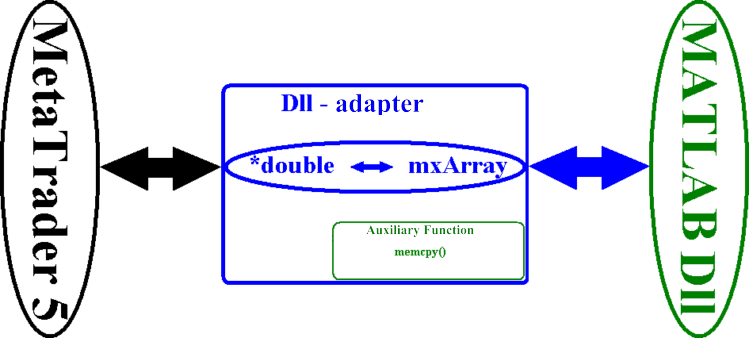It is very similar to the right side of DLL for MATLAB Engine, so we won't parse its algorithm and proceed directly to the code. To do this, create two small files in your C/C++ compiler:

nSMA.cpp (from DllMatlab.zip):

```#include <stdio.h>
#include <windows.h>
#include "mclmcr.h"
#include "NEOSMA.h"
/*---------------------------------------------------------------------------
** DLL Global Functions (external)
*/
extern "C" __declspec(dllexport) bool __stdcall IsStartSMA(void);
extern "C" __declspec(dllexport) bool __stdcall nSMA(double *pY,  int  nSizeY,
double *pIn, int nSizeIn,
/*---------------------------------------------------------------------------
** Global Variables
*/
mxArray *TempY;
mxArray *TempIn;
mxArray *TempN;
bool bIsNeoStart;
//---------------------------------------------------------------------------
int WINAPI DllEntryPoint(HINSTANCE hinst, unsigned long reason, void* lpReserved)
{
switch(reason)
{
case DLL_PROCESS_ATTACH:
bIsNeoStart = false;
TempY  = 0;   //Nullify pointers to buffers
TempN  = 0;
TempIn = 0;
break;
case DLL_PROCESS_DETACH:
NEOSMATerminate();
//Delete old data before exiting from Dll
if(TempY  != NULL) mxDestroyArray(TempY);
if(TempN  != NULL) mxDestroyArray(TempN);
if(TempIn != NULL) mxDestroyArray(TempIn);
mclTerminateApplication();
}
return 1;
}
//---------------------------------------------------------------------------
bool __stdcall IsStartSMA(void)
{
if(bIsNeoStart == false)
{
if(!mclInitializeApplication(NULL,0) )
{
MessageBoxA(NULL, (LPSTR)"Can't start MATLAB MCR!",
(LPSTR) "MATLAB DLL: ERROR!", MB_OK|MB_ICONSTOP);
return false;
}else
{
bIsNeoStart = NEOSMAInitialize();
};
};
return bIsNeoStart;
}
//---------------------------------------------------------------------------
bool __stdcall nSMA(double *pY, int nSizeY, double *pIn, int nSizeIn, double dN, double dAd)
{
/*
** Create buffers
*/
if(TempN == NULL){ TempN = mxCreateDoubleMatrix(1, 1, mxREAL);}
else
{
mxDestroyArray(TempN);
TempN= mxCreateDoubleMatrix(1, 1, mxREAL);
};
if(TempIn == NULL){ TempIn = mxCreateDoubleMatrix(1, nSizeIn, mxREAL);}
else
{
mxDestroyArray(TempIn);
TempIn= mxCreateDoubleMatrix(1, nSizeIn, mxREAL);
};
else
{
};
/*
** Creating data for processing
*/
memcpy((char *)mxGetPr(TempIn), (char *) pIn, (nSizeIn)*8);
memcpy((char *)mxGetPr(TempN), (char *) &dN, 8);
/*
** Send and receive a response from the m-function
*/
if(mlfNeoSMA(1, (mxArray **)TempY, (mxArray *)TempIn, (mxArray *)TempN
, (mxArray *)TempAd) == false) return false;
/*
** Return calculated vector from the m-function and clear buffers
*/
memcpy((char *) pY, (char *)mxGetPr(TempY), (nSizeY)*8);
mxDestroyArray((mxArray *)TempY);  TempY  = 0;
mxDestroyArray((mxArray *)TempN);  TempN  = 0;
mxDestroyArray((mxArray *)TempIn); TempIn = 0;
return true;
}
```

nSMA.def (from DllMatlab.zip):

LIBRARY nnSMA
EXPORTS
IsStartSMA
nSMA

Build the project in your C/C++ compiler: for this you need to include standard input/output library and add to project the following files (in your compiler: Project->Add Project):

1. nSMA.def
2. In <MATLAB>\Extern\lib\<win32/64>\<compiler>\ folder, where:
<MATLAB> — MATLAB main folder.
<win32/64> — either win32 folder for 32-bit OS, or win64 for 64-bit OS.
<compiler> — the "borland" folder for Borland C/C++ ver. 5-6, the "microsoft" folder for Microsoft Visual C++ (I have files for version 6):
• libmx.lib
• mclmcr.lib
3. NeoSMA.lib — create manually (see 3.1 Developing Universal Library of MetaTrader 5 & MATLAB Engine Interaction).

The last, what I want to tell you in this section, is about files needed when moving project to another computer, where there is no MATLAB installed.

Here is a list of files and paths on the target machine:

• MCRInstaller.exe                    any folder (MCR installer)
• extractCTF.exe                      any folder (for MCR installer)
• MCRRegCOMComponent.exe  any folder (for MCR installer)
• unzip.exe                              any folder (for MCR installer)
• NeoSMA.dll                           <terminal_dir>\MQL5\Libraries
• NeoSMA.ctf                           <terminal_dir>\MQL5\Libraries
• nnSMA.dll                             <terminal_dir>\MQL5\Libraries

Many advanced programmers have already guessed, that it is advisable to use an installer program (SETUP). There are many of them over the Internet, including free products.

Now we have to test this DLL in MetaTrader 5. To do this we will write a simple script (TestDllMatlab.mq5 from the DllMatlab.zip):

```#property copyright "2010, MetaQuotes Software Corp."
#property version   "1.00"
#import "nnSMA.dll"
bool  IsStartSMA(void);
bool  nSMA(double &pY[],
int nSizeY,
double &pIn[],
int nSizeIn,
double dN,
#import
datetime    Time[];    // dynamic array of time coordinates
double      Price[];   // dynamic array of price
double      dNeoSma[]; // dynamic array of price
void OnStart()
{
int ind=0;
// run Dll
if(IsStartSMA()==true)
{
//--- create and fill arrays
CopyTime(Symbol(),0,0,301,Time);   // time array + 1
ArraySetAsSeries(Time,true);       // get the time chart
CopyOpen(Symbol(),0,0,300,Price);  // price array
ArraySetAsSeries(Price,true);      // get the open prices
ArrayResize(dNeoSma,300,0);        // reserve space for function response
// get data
if(nSMA(dNeoSma,300,Price,300,1,2)==false) return;
// specify array orientation
ArraySetAsSeries(dNeoSma,true);
// plot data on chart
for(ind=0; ind<ArraySize(dNeoSma);ind++)
{
DrawPoint(IntegerToString(ind,5,'-'),Time[ind],dNeoSma[ind]);
}
}
}
//+------------------------------------------------------------------+
void DrawPoint(string NamePoint,datetime x,double y)
{  // 100% ready. Plot data on chart. Drawing using arrows.
// Main properties of chart object
ObjectCreate(0,NamePoint,OBJ_ARROW,0,0,0);
ObjectSetInteger(0, NamePoint, OBJPROP_TIME, x);        // time coordinate x
ObjectSetDouble(0, NamePoint, OBJPROP_PRICE, y);        // price coordinate y
// Additional properties of chart object
ObjectSetInteger(0, NamePoint, OBJPROP_WIDTH, 0);       // line width
ObjectSetInteger(0, NamePoint, OBJPROP_ARROWCODE, 173); // arrow type
ObjectSetInteger(0, NamePoint, OBJPROP_COLOR, Red);     // arrow color
}
//+------------------------------------------------------------------+
```

### Conclusion

Many readers may have a question: what method to choose? The answer is simple - both, because during the design/debugging of mathematical model the speed is not needed. But you'll need the full power of MATLAB without "special production costs" for programming. MATLAB Engine will help here, of course. However, when the mathematical model is debugged and ready to use, you'll need speed, multitasking (work of indicator and/or trade system at several price charts) - here without a doubt you'll need a DLL, built in MATLAB environment.

But all this does not oblige you to follow it. Everybody will give the answer to this question himself, relying primarily on the proportion of "programming cost" to the scale of the project (number of indicator and/or trade system users). It makes no sense to create Dll in the MATLAB environment for one or two users (it's easier to install MATLAB on two computers).

Many readers, who are unfamiliar with MATLAB, probably have a question: why all of this? MQL5 has already mathematical functions! The answer is that use of MATLAB enables you to effortlessly implement your mathematical ideas, here is just a partial list of possibilities:

• dynamic algorithm of fuzzy logic in the indicator and/or mechanical trade system
• dynamic genetic algorithm in mechanical trade system (dynamic strategy tester)
• dynamic neural network algorithm in the indicator and/or mechanical trade system
• three dimensional indicators
• simulation of nonlinear management systems

So, all in your hands, and do not forget: "Mathematics has always been the queen of sciences", and MATLAB package — is your scientific calculator.

### Literature

1. MATLAB built-in help.
2. MQL5 built-in help.
3. Jeffrey Richter. Programming Applications for Microsoft Windows.

Translated from Russian by MetaQuotes Software Corp.
Original article: https://www.mql5.com/ru/articles/44

Attached files |
dllmatlab_en.zip (955.7 KB)
matlabengine_en.zip (670.57 KB)
Last comments | Go to discussion (5)| 29 Aug 2010 at 18:56

I've followed your directions carefully but I'm unable to get this working with Matlab 2007b. I continuously get these errors, when trying to use your prebuilt examples, with the MT5 Terminal.

2010.08.29 10:33:30 TestMLEngine (EURUSD,H1) Cannot open 'C:\Users\QuantFX\AppData\Roaming\MetaQuotes\Terminal\216E2BD2BB8CE60176A41ADF6AC1C76D\MQL5\Libraries\LibMlEngine.dll' (126)

2010.08.29 10:33:47 TestDllMatlab (EURUSD,H1) Cannot open 'C:\Users\QuantFX\AppData\Roaming\MetaQuotes\Terminal\216E2BD2BB8CE60176A41ADF6AC1C76D\MQL5\Libraries\nnSMA.dll' (126)

I don't really know what to think of it becuase I'm able to get the project mentioned in the article   "How to Exchange Data: A DLL for MQL5 in 10 Minutes " found here https://www.mql5.com/en/articles/18 working without any problems.

2010.08.29 10:44:16 MQL5DLL_Test (EURUSD,H1) Access violation write to 0x00000000 in 'C:\Users\QuantFX\AppData\Roaming\MetaQuotes\Terminal\216E2BD2BB8CE60176A41ADF6AC1C76D\MQL5\Libraries\MQL5DLLSamples.dll'

2010.08.29 10:44:16 MQL5DLL_Test (EURUSD,H1) Replace: A quick brown cat jumps over the lazy dog

2010.08.29 10:44:16 MQL5DLL_Test (EURUSD,H1) Array: 0 1 2 3 4 5 6 7 8 9
2010.08.29 10:44:16 MQL5DLL_Test (EURUSD,H1) Time 297 msec, int: -752584127 double: 17247836076609

So the terminal obvioulsy has no problems with access violations to the \Libraries folder, and I'm certain the Expert Advisor "Options" allow for dll imports and autotrading...Any help would be greatly appreciated because I've been stumped by this since first reading this article. Thanks In Advance| 4 Sep 2010 at 08:44

Cheers| 9 Nov 2014 at 19:09

Hello,

Has anyone got 3.1 Developing Universal Library of MetaTrader 5 & MATLAB Engine Interaction works with Strategy Tester?

It works with standard expert advisor, but not with strategy tester. Can anybody point me where I may have done it wrong?| 11 Oct 2015 at 18:23

Will this work also for MT4 ??? Or it is exclusively setup for MT5 ??

Krzysztof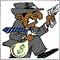| 6 Jul 2017 at 13:16

I found a little bug in UnitDll.cpp

Its better to check  with OR and not AND

So you cant send a command to a closed Engine

```bool __stdcall mlxInputChar(char *CharArray)
{//100% ready. Function of passing command/function/text to desktop
/*
** Check if virtual machine and string are present
*/
----> // Original with Bug -> if((pEng == NULL)&&(strlen(CharArray)<1)) return false;        <---
if ((pEng == NULL) || (strlen(CharArray)<1)) return false;
/*
** Enter formula into MATLAB desktop
*/
engEvalString((Engine *)pEng, CharArray);
return true;
}```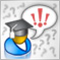Limitations and Verifications in Expert Advisors

Is it allowed to trade this symbol on Monday? Is there enough money to open position? How big is the loss if Stop Loss triggers? How to limit the number of pending orders? Was the trade operation executed at the current bar or at the previous one? If a trade robot cannot perform this kind of verifications, then any trade strategy can turn into a losing one. This article shows the examples of verifications that are useful in any Expert Advisor.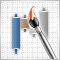Creating Multi-Colored Indicators in MQL5

In this article, we will consider how to create multi-colored indicators or convert the existing ones to multi-color. MQL5 allows to represent the information in the convenient form. Now it isn't necessary to look at a dozen of charts with indicators and perform analyses of the RSI or Stochastic levels, it's better just to paint the candles with different colors depending on the values of the indicators.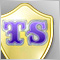How to Create Your Own Trailing Stop

The basic rule of trader - let profit to grow, cut off losses! This article considers one of the basic techniques, allowing to follow this rule - moving the protective stop level (Stop loss level) after increasing position profit, i.e. - Trailing Stop level. You'll find the step by step procedure to create a class for trailing stop on SAR and NRTR indicators. Everyone will be able to insert this trailing stop into their experts or use it independently to control positions in their accounts.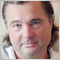Interview with Leonid Velichkovsky: "The Biggest Myth about Neural Networks is Super-Profitability" (ATC 2010)

The hero of our interview Leonid Velichkovski (LeoV) has already participated in Automated Trading Championships. In 2008, his multicurrency neural network was like a bright flash in the sky, earning \$110,000 in a certain moment, but eventually fell victim to its own aggressive money management. Two years ago, in his interview Leonid share his own trading experience and told us about the features of his Expert Advisor. On the eve of the ATC 2010, Leonid talks about the most common myths and misconceptions associated with neural networks.